# 逻辑回归概述+逻辑回归的优点+sklearn中的逻辑回归算法+逻辑回归中的特征工程+梯度下降+二元回归与多元回归+样本不平衡与参数class_weight1512-高同学

, ,

# 1、逻辑回归概述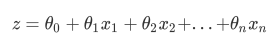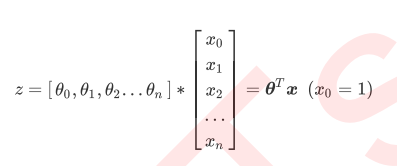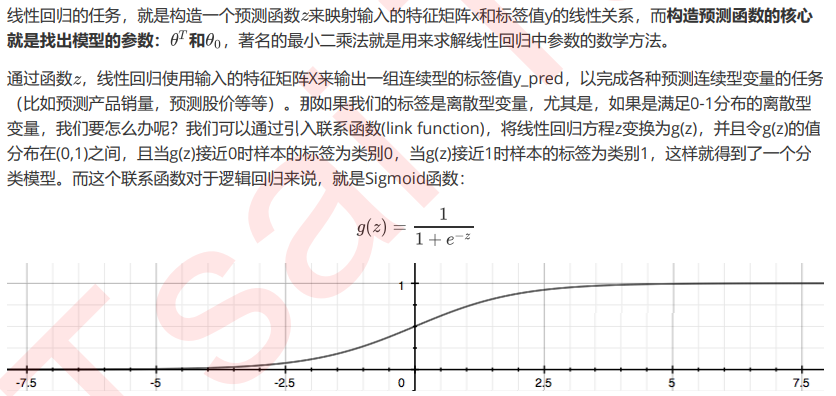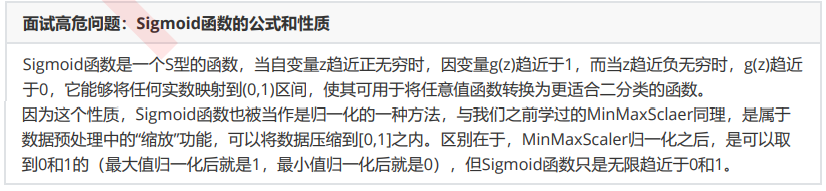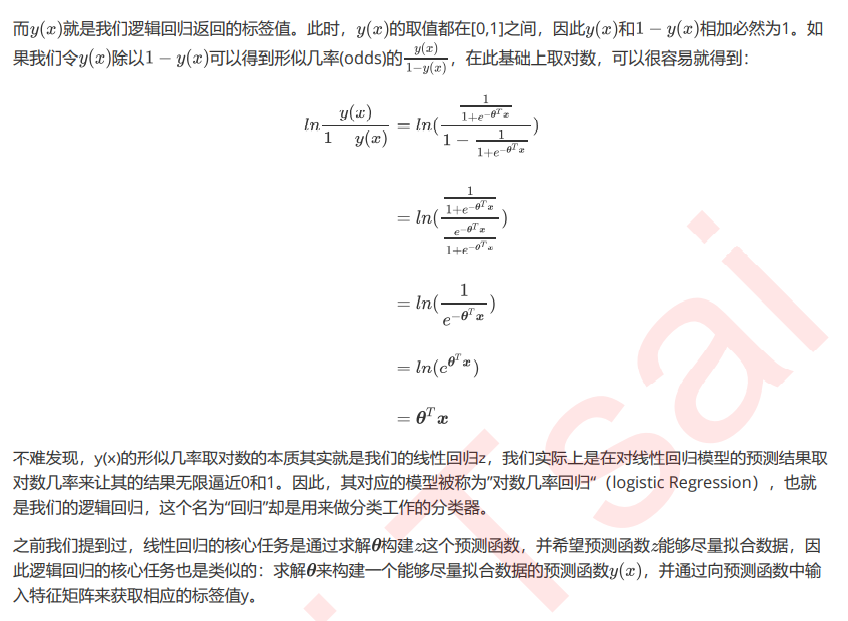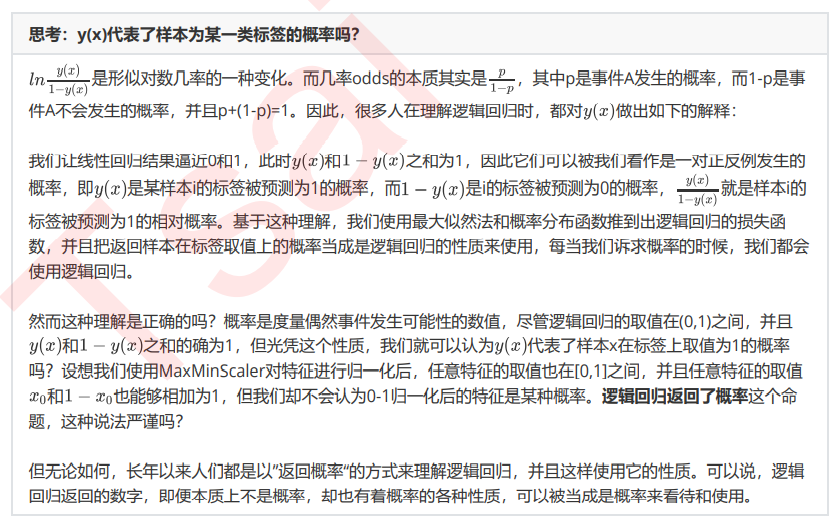# 2、为什么需要逻辑回归？

（1）逻辑回归对线性关系的拟合效果好到丧心病狂，特征与标签之间的线性关系极强的数据，比如金融领域中的 信用卡欺诈，评分卡制作，电商中的营销预测等等相关的数据，都是逻辑回归的强项。虽然现在有了梯度提 升树GDBT，比逻辑回归效果更好，也被许多数据咨询公司启用，但逻辑回归在金融领域，尤其是银行业中的 统治地位依然不可动摇（相对的，逻辑回归在非线性数据的效果很多时候比瞎猜还不如，所以如果你已经知 道数据之间的联系是非线性的，千万不要迷信逻辑回归）；

（2） 逻辑回归计算快：对于线性数据，逻辑回归的拟合和计算都非常快，计算效率优于SVM和随机森林，亲测表示在大型数据上尤其能够看得出区别；

（3）**逻辑回归返回的分类结果不是固定的0，1，而是以小数形式呈现的类概率数字：**我们因此可以把逻辑回归返 回的结果当成连续型数据来利用。比如在评分卡制作时，我们不仅需要判断客户是否会违约，还需要给出确 定的”信用分“，而这个信用分的计算就需要使用类概率计算出的对数几率，而决策树和随机森林这样的分类 器，可以产出分类结果，却无法帮助我们计算分数（当然，在sklearn中，决策树也可以产生概率，使用接口 predict_proba调用就好，但一般来说，正常的决策树没有这个功能）。

# 3、sklearn中的逻辑回归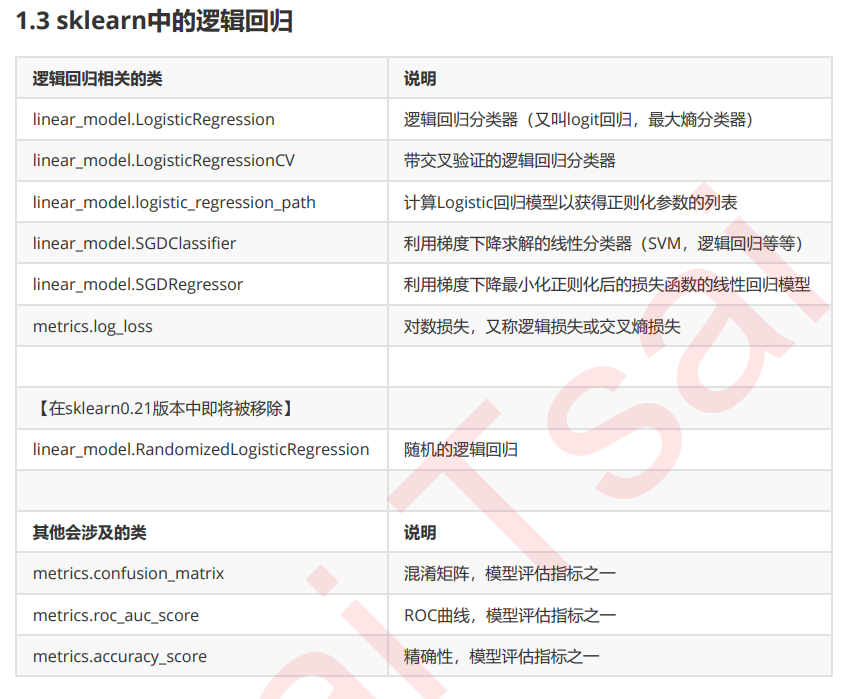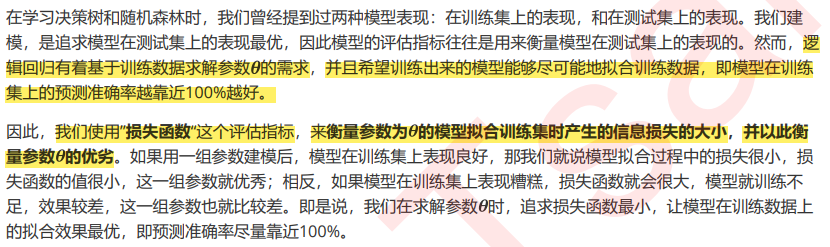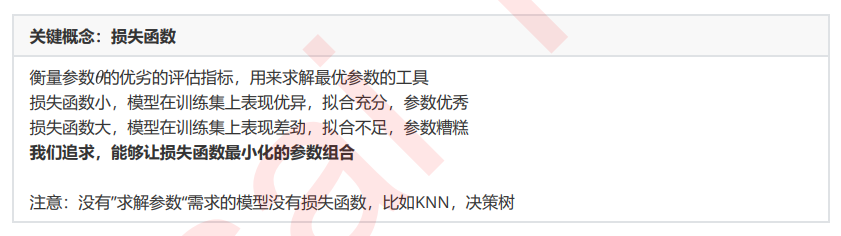# 4、损失函数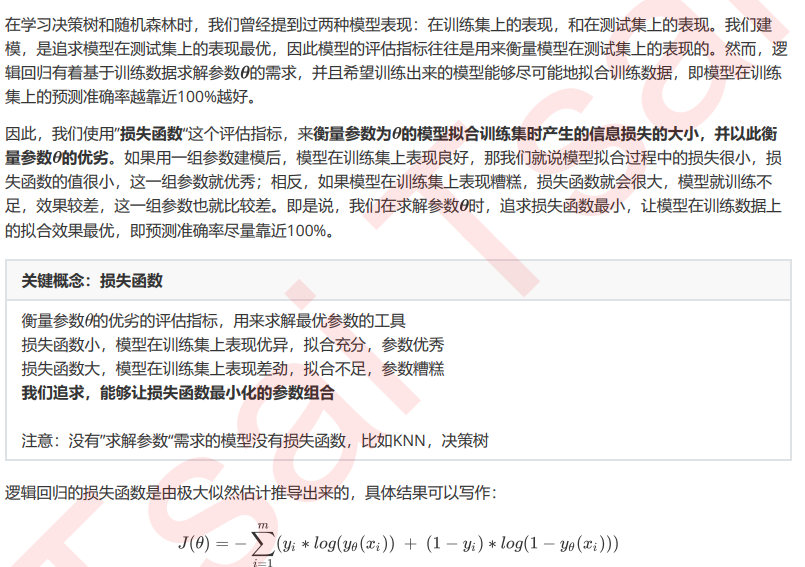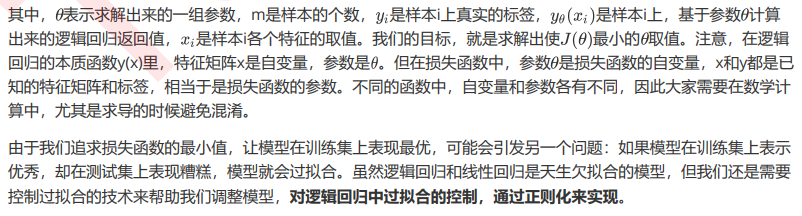# 5、重要参数penatly&C

（1）正则化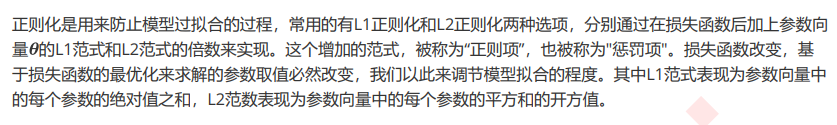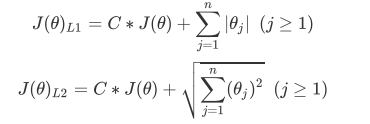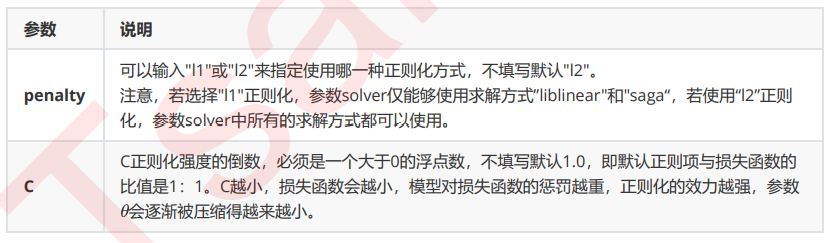L1正则化和L2正则化虽然都可以控制过拟合，但它们的效果并不相同。当正则化强度逐渐增大（即C逐渐变小）， 参数的取值会逐渐变小，但L1正则化会将参数压缩为0，L2正则化只会让参数尽量小，不会取到0。

from sklearn.datasets import load_breast_cancer
from sklearn.metrics import accuracy_score
from sklearn.linear_model import LogisticRegression as LR
from sklearn.model_selection import train_test_split
import numpy as np
import matplotlib.pyplot as plt

#print(data)
x = data.data
y = data.target

#print(x)
#print('###########################33')
#print(y)

lr1 = LR(penalty='l1', solver='liblinear', C=0.5, max_iter=1000)
lr2 = LR(penalty='l2', solver='liblinear', C=0.5, max_iter=1000)

#逻辑回归的重要属性coef_，查看每个特征对应的参数
lrl1 = lr1.fit(x, y)
#print(lrl1.coef_)
#print((lrl1.coef_ != 0).sum()) #10

lrl2 = lr2.fit(x, y)
#print(lrl2.coef_)
#print((lrl2.coef_ != 0).sum())  #30

l1 = []
l2 = []
l1test = []
l2test = []

xtrain, xtest, ytrain, ytest = train_test_split(x, y, test_size=0.3, random_state=0)

for i in np.linspace(0.5, 1, 19):
lrl1 = LR(penalty='l1', solver='liblinear', C=i, max_iter=1000)
lrl2 = LR(penalty='l2', solver='liblinear', C=i, max_iter=1000)

lrl1 = lrl1.fit(xtrain, ytrain)
l1.append(accuracy_score(lrl1.predict(xtrain), ytrain))
l1test.append(accuracy_score(lrl1.predict(xtest), ytest))

lrl2 = lrl2.fit(xtrain, ytrain)
l2.append(accuracy_score(lrl2.predict(xtrain), ytrain))
l2test.append(accuracy_score(lrl2.predict(xtest), ytest))

graph = [l1, l2, l1test, l2test]
color = ['green', 'black', 'red', 'blue']
label = ['L1', 'L2', 'L1test', 'L2test']

plt.figure(figsize=(10, 8))
for i in range(4):
plt.plot(np.linspace(0.5, 1, 19), graph[i], color[i], label=label[i])

plt.legend(loc=4)
plt.show()


# 6、逻辑回归中的特征工程

## 高效的嵌入法embedded

from sklearn.linear_model import LogisticRegression as LR
from sklearn.model_selection import cross_val_score
from sklearn.feature_selection import  SelectFromModel
import numpy as np
import matplotlib.pyplot as plt

x = data.data
y = data.target
#print(x.shape)  #(569, 30) 有30个特征
#print(y.shape)  #(569,)

LR_ = LR(solver='liblinear', C=0.9, random_state=0)
score = cross_val_score(LR_, x, y, cv=10).mean()  #0.9508145363408522
#print(score)

#x_embedded = SelectFromModel(LR_, norm_order=1).fit_transform(x, y)
#print(x_embedded.shape)  #(569, 9)  #降到9维了

coef_list = LR_.fit(x, y).coef_
coef_abs = abs((coef_list))
coef_max = coef_abs.max()
#print(coef_max) #1.9937460483274254

fullx = []
fsx = []
threshold = np.linspace(0, abs((LR_.fit(data.data,data.target).coef_)).max(), 20)
k = 0

for i in threshold:
X_embedded = SelectFromModel(LR_, threshold=i).fit_transform(data.data, data.target)
fullx.append(cross_val_score(LR_, data.data, data.target, cv=5).mean())
fsx.append(cross_val_score(LR_, X_embedded, data.target, cv=5).mean())
print((threshold[k], X_embedded.shape))
k += 1

plt.figure(figsize=(20, 5))
plt.plot(threshold, fullx, label="full")
plt.plot(threshold, fsx, label="feature selection")
plt.xticks(threshold)
plt.legend()
plt.show()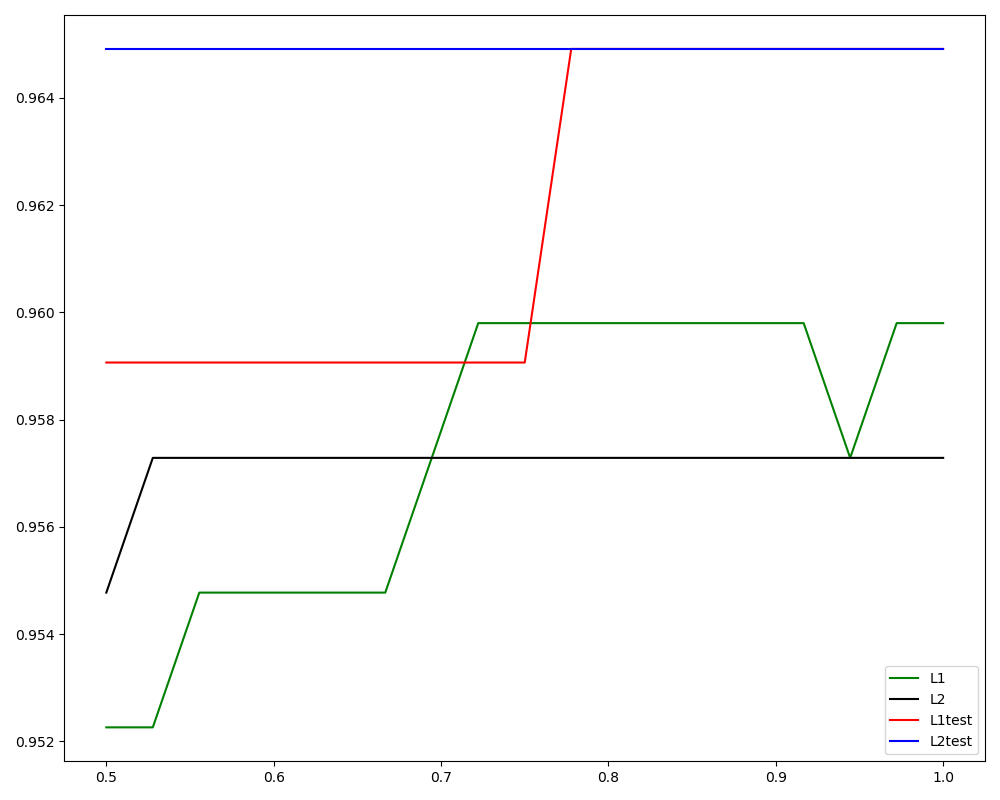for i in threshold:
X_embedded = SelectFromModel(LR_, threshold=i).fit_transform(data.data, data.target)
score_1 = cross_val_score(LR_, data.data, data.target, cv=5).mean()
fullx.append(score_1)
score_2 = cross_val_score(LR_, X_embedded, data.target, cv=5).mean()
fsx.append(score_2)
print((threshold[k], X_embedded.shape))
k += 1

plt.figure(figsize=(20, 5))
plt.plot(threshold, fullx, label="full")
plt.plot(threshold, fsx, label="feature selection")
plt.xticks(threshold)
plt.legend()
plt.show()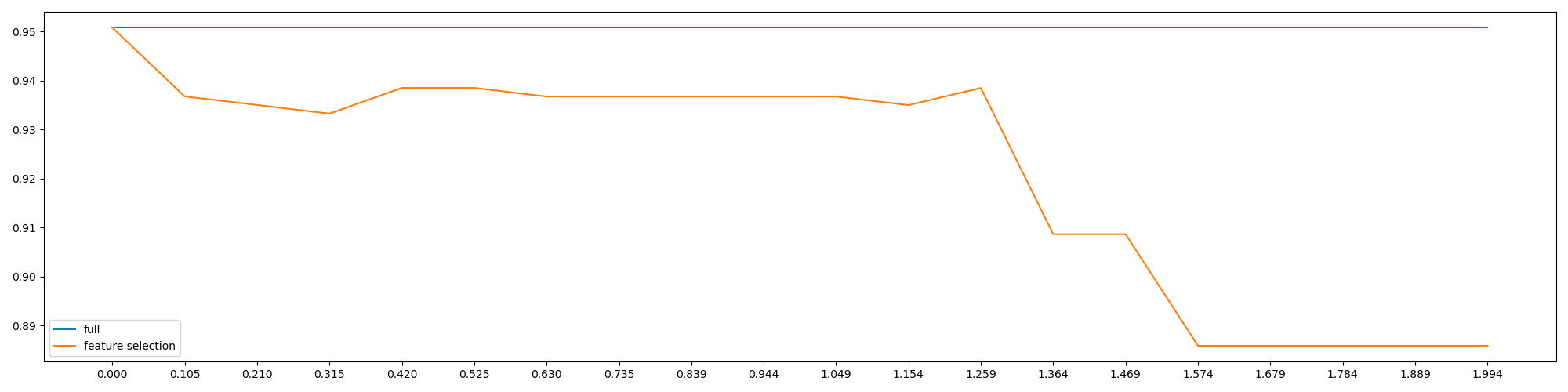fullx = []
fsx = []
C = np.arange(0.01, 10.01, 0.5)
#rint(C)

for i in C:
LR_ = LR(solver='liblinear', C=i, random_state=0)
score_1 = cross_val_score(LR_, x, y, cv=10).mean()
fullx.append(score_1)
x_embedded = SelectFromModel(LR_, norm_order=1).fit_transform(x, y)
score_2 = cross_val_score(LR_, x_embedded, y, cv=10).mean()
fsx.append(score_2)

print(max(fsx), C[fsx.index(max(fsx))])
#0.9543546365914788 5.01

plt.figure(figsize=(20, 5))
plt.plot(C, fullx, label='full')
plt.plot(C, fsx, label='feature selection')
plt.xticks(C)
plt.legend()
plt.show()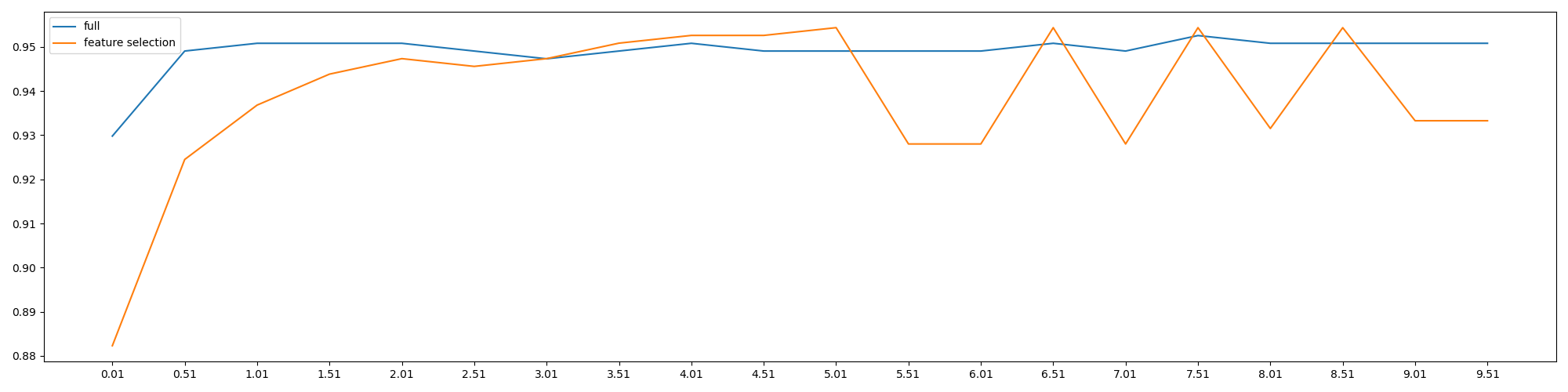fullx = []
fsx = []
C = np.arange(3.5, 4.5, 0.005)

for i in C:
LR_ = LR(solver='liblinear', C=i, random_state=0)
score_1 = cross_val_score(LR_, x, y, cv=10).mean()
fullx.append(score_1)
x_embedded = SelectFromModel(LR_, norm_order=1).fit_transform(x, y)
score_2 = cross_val_score(LR_, x_embedded, y, cv=10).mean()
fsx.append(score_2)

print(max(fsx), C[fsx.index(max(fsx))])
#0.9543546365914788 3.6049999999999978
plt.figure(figsize=(25, 5))
plt.plot(C, fullx, label='full')
plt.plot(C, fsx, label='feature selection')
plt.xticks(C)
plt.legend()
plt.show()


LR_ = LR(solver='liblinear', C=3.6049999999999978, random_state=0)
print(cross_val_score(LR_, x, y, cv=10).mean())
#0.9473057644110275

LR_ = LR(solver='liblinear', C=3.6049999999999978, random_state=0)
x_embedded = SelectFromModel(LR_, norm_order=1).fit_transform(x, y)
print(cross_val_score(LR_, x_embedded, y, cv=10).mean())
#0.9543546365914788



# 7、梯度下降

## （1）梯度下降的概念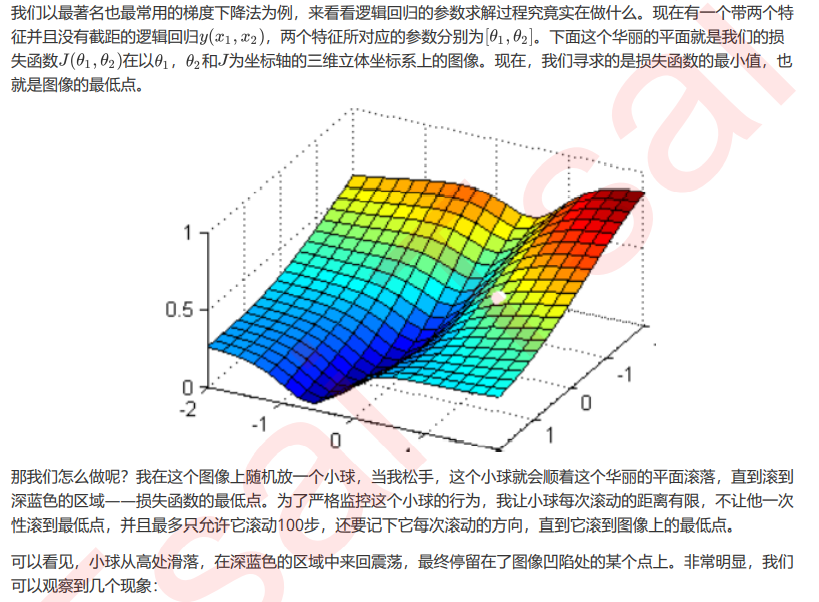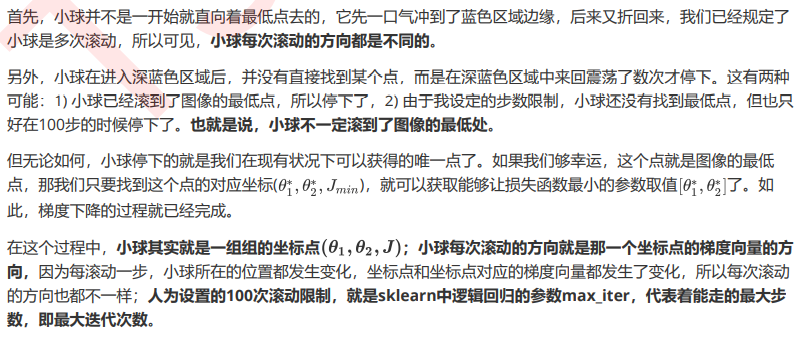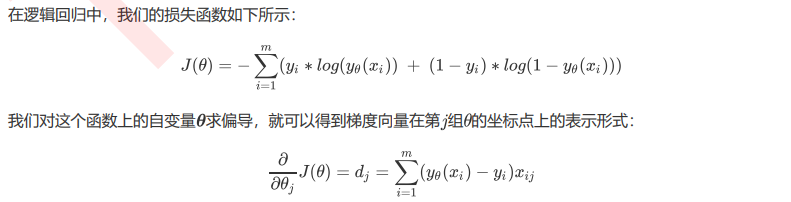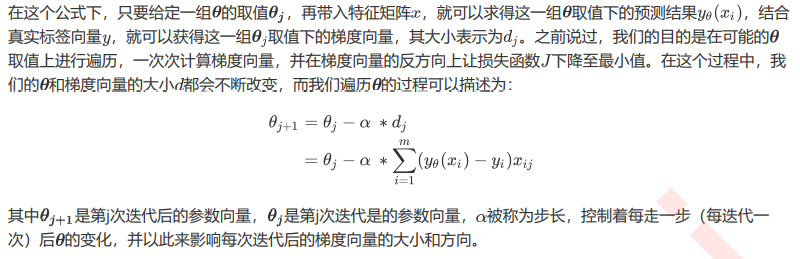## （2）步长的概念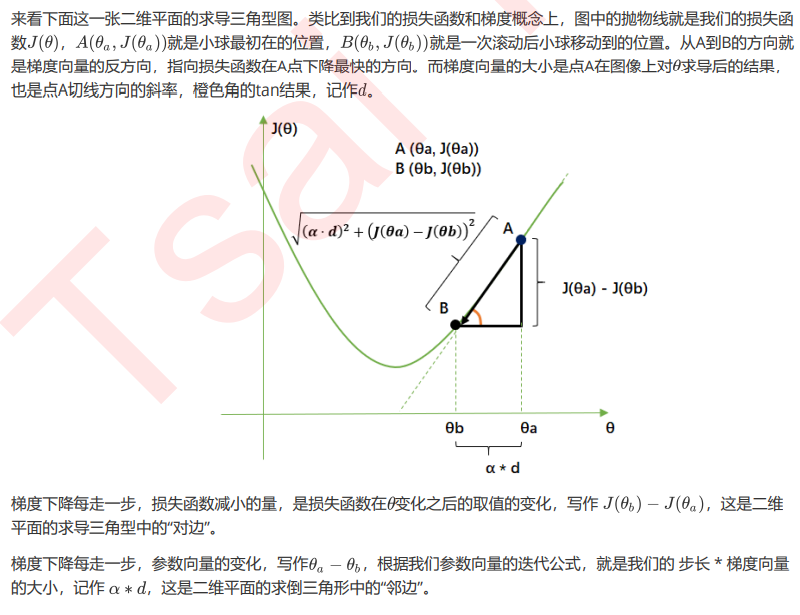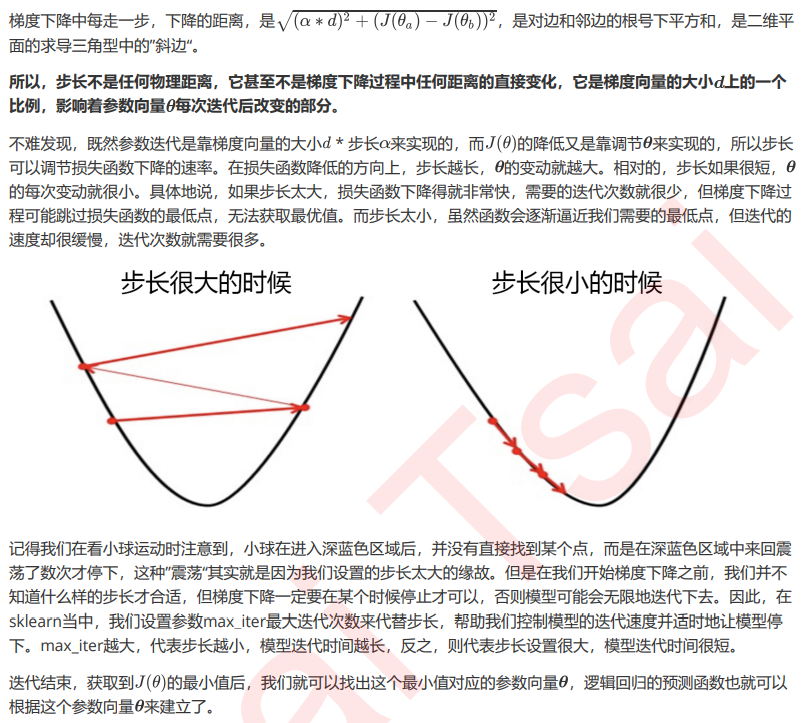max_iter学习曲线

from sklearn.datasets import load_breast_cancer
from sklearn.model_selection import train_test_split
from sklearn.metrics import accuracy_score
from sklearn.linear_model import LogisticRegression as LR
from matplotlib import  pyplot as plt
import numpy as np

x = data.data
y = data.target
#print(x.shape)  #(569, 30)
#print(y.shape)  #(569,)

xtrain, xtest, ytrain, ytest = train_test_split(x, y, test_size=0.3, random_state=0)

lr1 = []
lr1test = []
for i in range(1, 201, 10):
LR_ = LR(penalty='l1', solver='liblinear', C=0.8, max_iter=i)
lr = LR_.fit(xtrain, ytrain)
score_1 = accuracy_score(lr.predict(xtrain), ytrain)
lr1.append(score_1)
l1test = LR_.fit(xtest, ytest)
score_2 = accuracy_score(lr.predict(xtest), ytest)
lr1test.append(score_2)

graph = [lr1, lr1test]
color = ['black', 'red']
label = ['l1', 'l1test']
plt.figure(figsize=(20, 5))
for i in range(2):
plt.plot(np.arange(1, 201, 10), graph[i], color[i], label=label[i])
plt.legend()
plt.xticks(np.arange(1, 201, 10))
plt.show()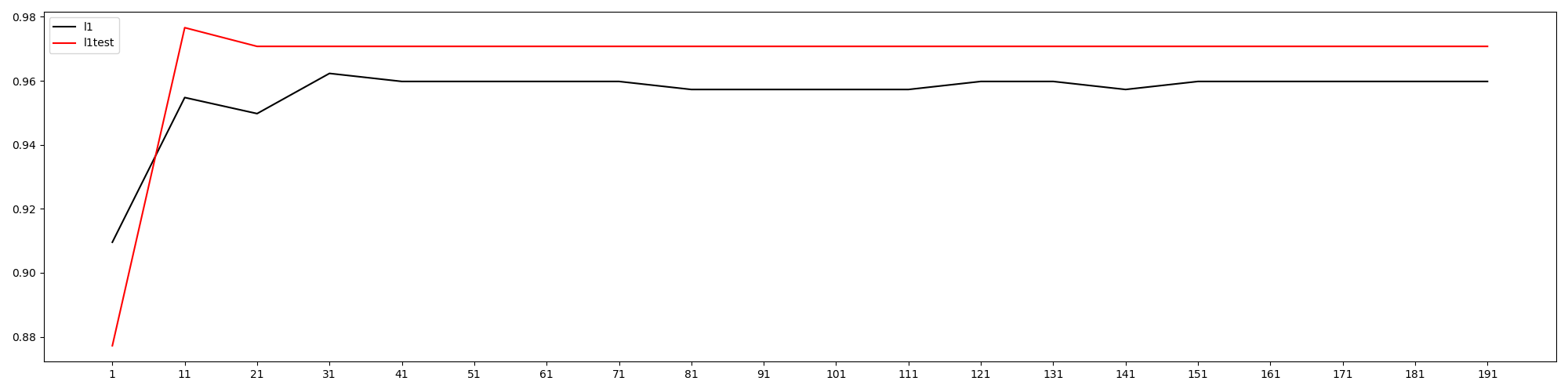# 8、二元回归与多元回归：重要参数solver & multi_class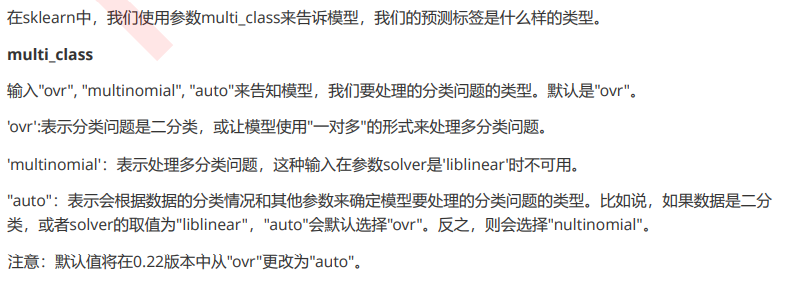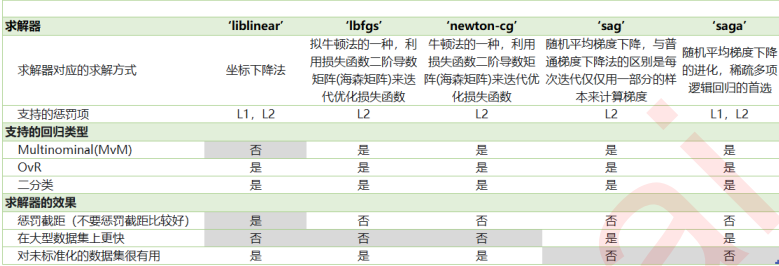from sklearn.datasets import load_iris
from sklearn.linear_model import LogisticRegression as LR
import numpy as np

x = data.data
y = data.target

for i in ('multinomial', 'ovr'):
clf = LR(solver='sag', max_iter=100, random_state=42, multi_class=i).fit(x, y)
print("training score : %.3f (%s)" % (clf.score(x, y), i))
#training score : 0.987 (multinomial)
#training score : 0.960 (ovr)


## 9、样本不平衡与参数class_weight

### 评论 抢沙发### 觉得文章有用就打赏一下文章作者

#### 支付宝扫一扫打赏#### 微信扫一扫打赏Vieu3.3主题Q Q 登 录